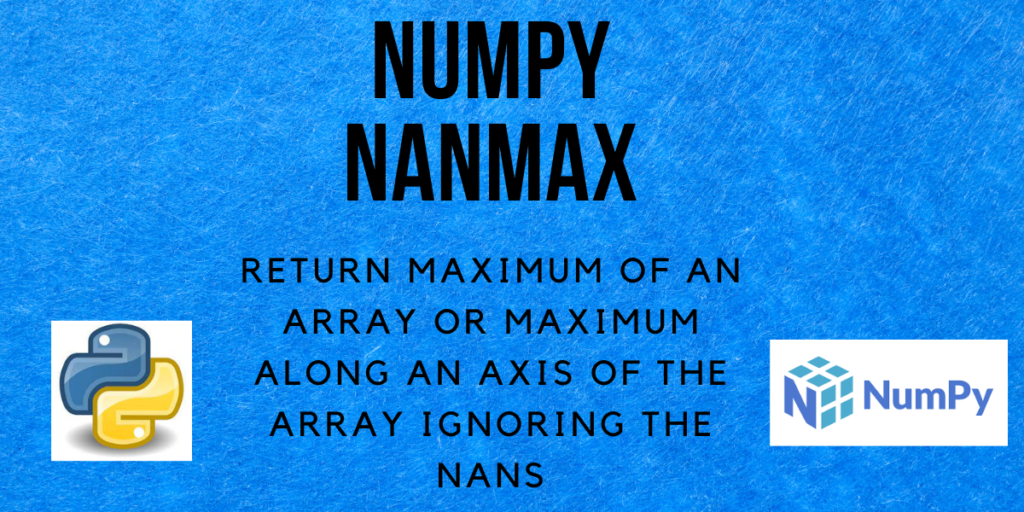# NumPy nanmax – Maximum of an array along an axis ignoring any NaNsHello and welcome to this tutorial on Numpy nanmax. In this tutorial, we will be learning about the NumPy `nanmax()` method and also seeing a lot of examples regarding the same. So let us begin!

Also read: NumPy amax – Maximum of an array of maximum along an axis

## What is NumPy nanmax?

In Python, NaN denotes Not a Number. If we have an array that contains some NaN values and want to find the maximum value in it, we can use the `nanmax()` method from NumPy.

The `nanmax()` method in NumPy is a function that returns the maximum of the array elements calculated by ignoring the NaN values in the array. It can be the maximum of all the array elements, the maximum of the array elements along the rows, or the maximum of the array elements along the columns.

We will see the examples for each of these in the upcoming section of this tutorial.

## Syntax of NumPy nanmax

Let us have a look at the syntax of the `nanmax()` function.

```numpy.nanmax(a, axis=None, out=None, keepdims=<no value>, initial=<no value>, where=<no value>)
```

Returns:
An array containing the maximum of the array along the specified axis, ignoring all the NaNs.

## Examples of NumPy nanmax

Let’s get right into the different examples of using numpy.nanmax() function.

### Nanmax of a 1-d array

```import numpy as np

arr = [5, 32, 10, np.nan, 4]
# using the nanmax function to calculate the maximum
ans = np.nanmax(arr)

print("arr =", arr)
print("Result =", ans)
```

Output:

```arr = [5, 32, 10, nan, 4]
Result = 32.0
```

Ignoring the NaN value, the maximum value among 5, 32, 10 and 4 is 32, hence it is returned.

### Nanmax of a 2-d array

```import numpy as np

arr = [[-12, 3], [np.nan, 36]]
# using the nanmax function to calculate the maximum
ans = np.nanmax(arr)

print("arr =", arr)
print("Result =", ans)
```

Output:

```arr = [[-12, 3], [nan, 36]]
Result = 36.0
```

Similar to the previous example, the maximum of -12, 3, and 36 is 36.

### Nanmax along an axis of the array

#### axis = 0

```import numpy as np

arr = [[5, 8], [np.nan, 36]]
# using the nanmax function to calculate the maximum
ans = np.nanmax(arr, axis=0)

print("arr =", arr)
print("Result =", ans)
```

Output:

```arr = [[5, 8], [nan, 36]]
Result = [ 5. 36.]
```

Here, the values in each row for a specific column are compared to find the maximum element.

```ans = max(arr, arr) = max(5, np.nan) = 5 (ignoring NaN)
ans = max(arr, arr) = max(8, 36) = 36
```

#### axis = 1

```import numpy as np

arr = [[5, 8], [np.nan, 36]]
# using the nanmax function to calculate the maximum
ans = np.nanmax(arr, axis=1)

print("arr =", arr)
print("Result =", ans)
```

Output:

```arr = [[5, 8], [nan, 36]]
Result = [ 8. 36.]
```

When axis=1, the elements in each row are compared over all the columns to find the maximum.

```ans = max(arr, arr) = max(5, 8) = 8
ans = max(arr, arr) = max(np.nan, 36) = 36 (ignoring NaN)
```

### NumPy nanmax of an array containing infinity

Let us now see how the `numpy.nanmax()` method handles infinity along with NaNs in the array.

```import numpy as np

# array containing +infinity
a = np.array([25, np.nan, 36, np.inf, 8])
# array containing -infinity
b = np.array([25, np.nan, 36, np.NINF, 8])
# array containing +infinity and -infinity
c = np.array([25, np.nan, 36, np.inf, np.NINF, 8])

max_a = np.nanmax(a)
max_b = np.nanmax(b)
max_c = np.nanmax(c)

print("a =", a)
print("Maximum of the array a =", max_a)
print("\nb =", b)
print("Maximum of the array b =", max_b)
print("\nc =", c)
print("Maximum of the array c =", max_c)

```

Output:

```a = [25. nan 36. inf  8.]
Maximum of the array a = inf

b = [ 25.  nan  36. -inf   8.]
Maximum of the array b = 36.0

c = [ 25.  nan  36.  inf -inf   8.]
Maximum of the array c = inf
```

In the above code, NINF denotes -infinity and inf denotes infinity. Note that,

• If the array contains positive infinity then the maximum is positive infinity.
• If the array contains negative infinity then the maximum is the maximum of all the elements, ignoring the NaNs.
• If the array contains both positive and negative infinity, then the maximum of the array is inf, i.e. positive infinity.

## Summary

That’s all! In this tutorial, we learned about the Numpy nanmax method and practiced different types of examples using the same.